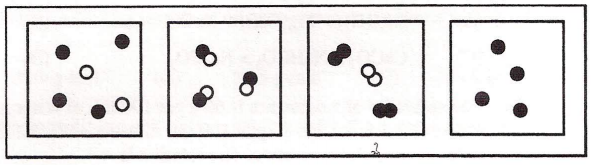# Problem: Which diagrams represent the pure substances?(A) 1 and 2(B) 1 and 3(C) 2 and 3(D) 2 and 4

###### FREE Expert Solution
79% (198 ratings)
###### Problem Details

Which diagrams represent the pure substances?

(A) 1 and 2

(B) 1 and 3

(C) 2 and 3

(D) 2 and 4What scientific concept do you need to know in order to solve this problem?

Our tutors have indicated that to solve this problem you will need to apply the Classification of Matter concept. You can view video lessons to learn Classification of Matter. Or if you need more Classification of Matter practice, you can also practice Classification of Matter practice problems.

What is the difficulty of this problem?

Our tutors rated the difficulty ofWhich diagrams represent the pure substances?(A) 1 and 2(B) ...as low difficulty.

How long does this problem take to solve?

Our expert Chemistry tutor, Sabrina took 1 minute and 22 seconds to solve this problem. You can follow their steps in the video explanation above.

What professor is this problem relevant for?

Based on our data, we think this problem is relevant for Professor Sarasua's class at UWF.